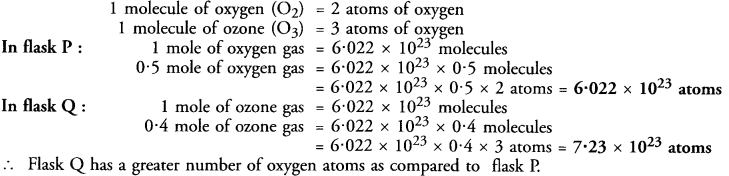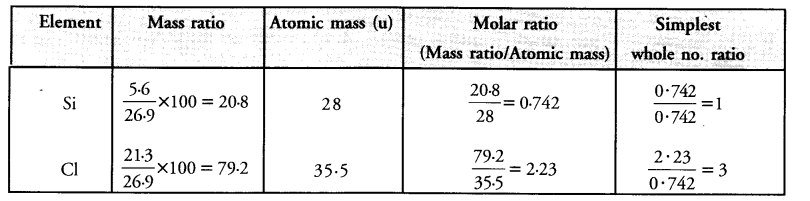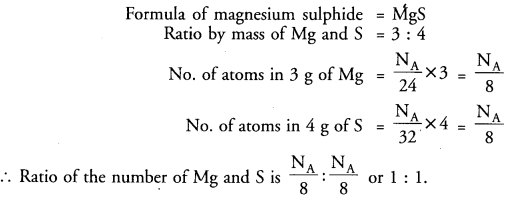## Online Education HOTS Questions for Class 9 Science Chapter 3 Atoms and Molecules

These Solutions are part of Online Education HOTS Questions for Class 9 Science. Here we have given HOTS Questions for Class 9 Science Chapter 3 Atoms and Molecules

Question 1.
(a) An element shows variable valencies 4 and 6. Write the formulae of its two oxides.
(b) An element forms an oxide A2O5.
(i) What is the valency of the element A ?
(ii) What will be the formula of the chloride of the element ?
(a) Let the element be represented by the symbol E.
Formula of oxide in which valency of E is 4 = E2O4 or EO2
Formula of oxide in which valency of E is 6 = E2O6 or EO3
(b) Formula of oxide of the element = A2O5
(i) The valency of the element A in the oxide = 5+
(ii) The formula of the chloride of the element A = ACl5.

More Resources

Question 2.
On analysing an impure sample of sodium chloride, the percentage of chlorine was found to be 45.5 What is the percentage of pure sodium chloride in the sample ?Question 3.
(a) Why does not the atomic mass of an element represent the actual mass of its atom ?
(b) The atomic mass of an element is in fraction. What does it mean ?
(c) Why is the value of Avogadro’s number 6.022 x 1023 and not any other value ?
(d) Does one gram mole of a gas occupy 24.4 L under all conditions of temperature and pressure ?
(a) Atoms of different elements are very small in size and their actual mass are extremely small. For example, the mass of an atom of hydrogen is 1.67 x 10-27 kg. To solve this problem, we consider the relative atomic masses of the elements. The relative atomic mas of hydrogen is 1 u and its corresponding gram atomic mass is 1 g.
(b) If the atomic mass of an element is in fraction, this means that it exists in the form of isotopes. The atomic mass is the average atomic mass and is generally fractional.
(c) It represents the number of atoms in one gram atom of an element or the number of molecules in one gram mole of a compound. If we divide the atomic mass of an element by actual mass of its atom, the value is 6.022 x 1023. Similarly, by dividing the molecular mass of a compound by the actual mass of its molecule, the same result is obtained.
(d) No, one gram mole of a gas occupies a volume of 22.4 L only under N.T.P. conditions i.e. at 273 K temperature and under 760 mm pressure.

Question 4.
A flask P contains 0.5 g mole of oxygen gas. Another flask Q contains 0.4 mole of ozone gas. Which of the two flasks contains greater number of oxygen atoms ?Question 5.
What weight of calcium contains the same number of atoms as are present in 3.2 g of sulphur ?Question 6.
Silicon forms a compound with chlorine in which 5.6 g of silicon is combined with 21.3 g of chlorine..
Calculate the formula of the compound (Atomic mass : Si = 28 : Cl = 35-5).